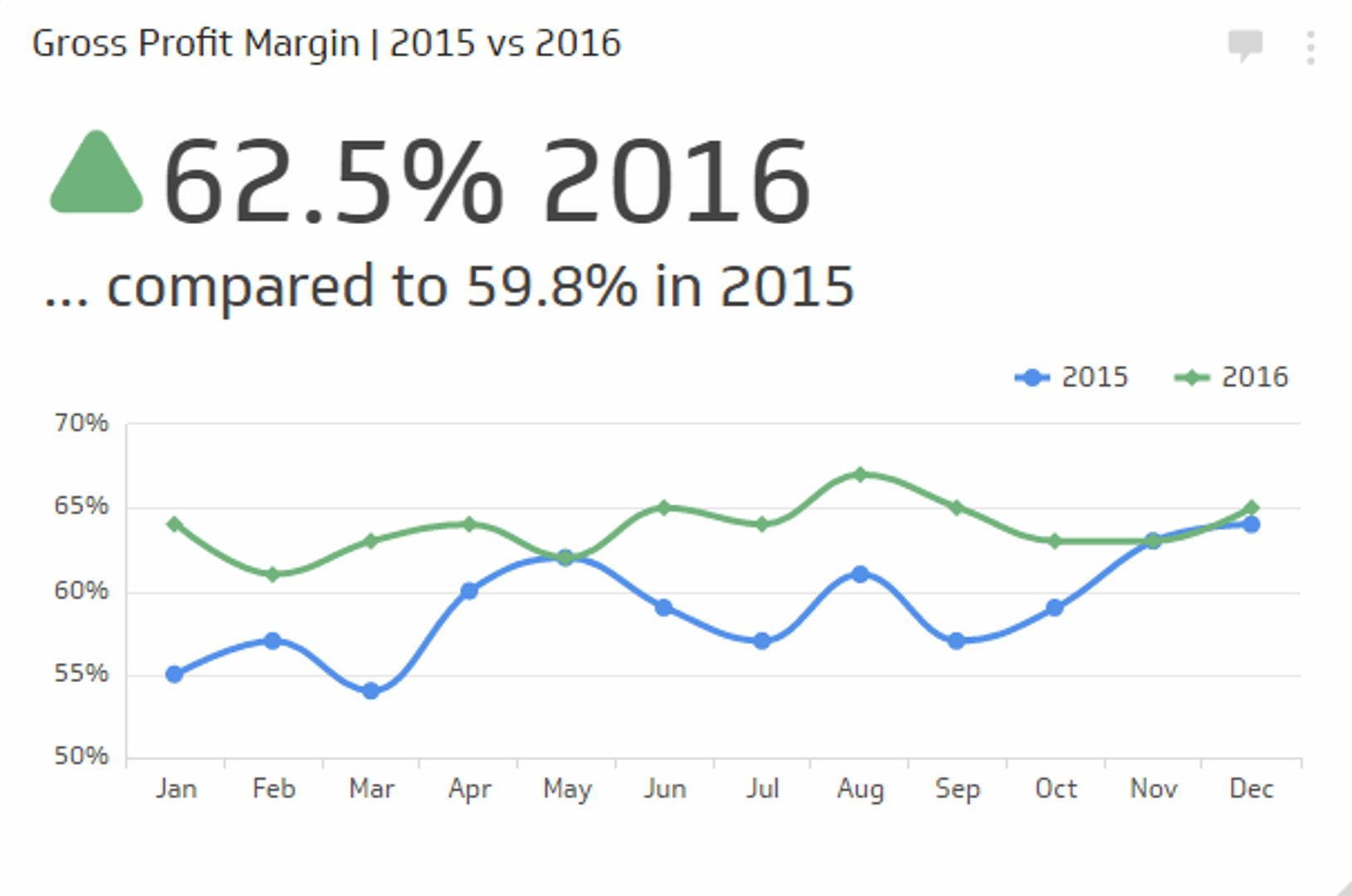# Gross Profit Margin

Measures how much profit you make on each dollar of sales before expenses.

## Track all your Financial KPIs in one place## What is Gross Profit Margin?

The Gross Profit Margin measures how much profit you make on each dollar of sales before expenses. This ratio is calculated by looking at the difference between production costs (excluding overhead, payroll, and taxes). It is important to note that gross profit margin isn't a true indicator of whether your business is marking a profit – for that, you will need to refer to another financial KPI: Net Profit Margin.

## Gross Profit Formula

Gross Profit Margin = (Gross Profit / Revenue) x 100

Where:

• Gross Profit is the total revenue minus the cost of goods sold (COGS).
• Revenue refers to the total income generated from the sale of goods or services before any expenses are deducted.

The formula demonstrates the proportion of each dollar earned that remains as profit after accounting for the cost of goods sold.

Note: Gross Profit and Total Revenue can be found on the income statement.

## Examples of Gross Profit Margin

To better understand the concept of Gross Profit Margin, let's examine some examples.

### Example 1:

A clothing retailer has the following financial information:

• Revenue: \$1,000,000
• Cost of Goods Sold: \$600,000

Using the Gross Profit Margin formula:

Gross Profit Margin = ((\$1,000,000 - \$600,000) / \$1,000,000) x 100 = 40%

This means that for every dollar of revenue, the retailer retains \$0.40 as gross profit.

### Example 2:

A software company has the following financial information:

• Revenue: \$2,500,000
• Cost of Goods Sold: \$1,000,000

Using the Gross Profit Margin formula:

Gross Profit Margin = ((\$2,500,000 - \$1,000,000) / \$2,500,000) x 100 = 60%

This indicates that for every dollar of revenue, the software company retains \$0.60 as gross profit.